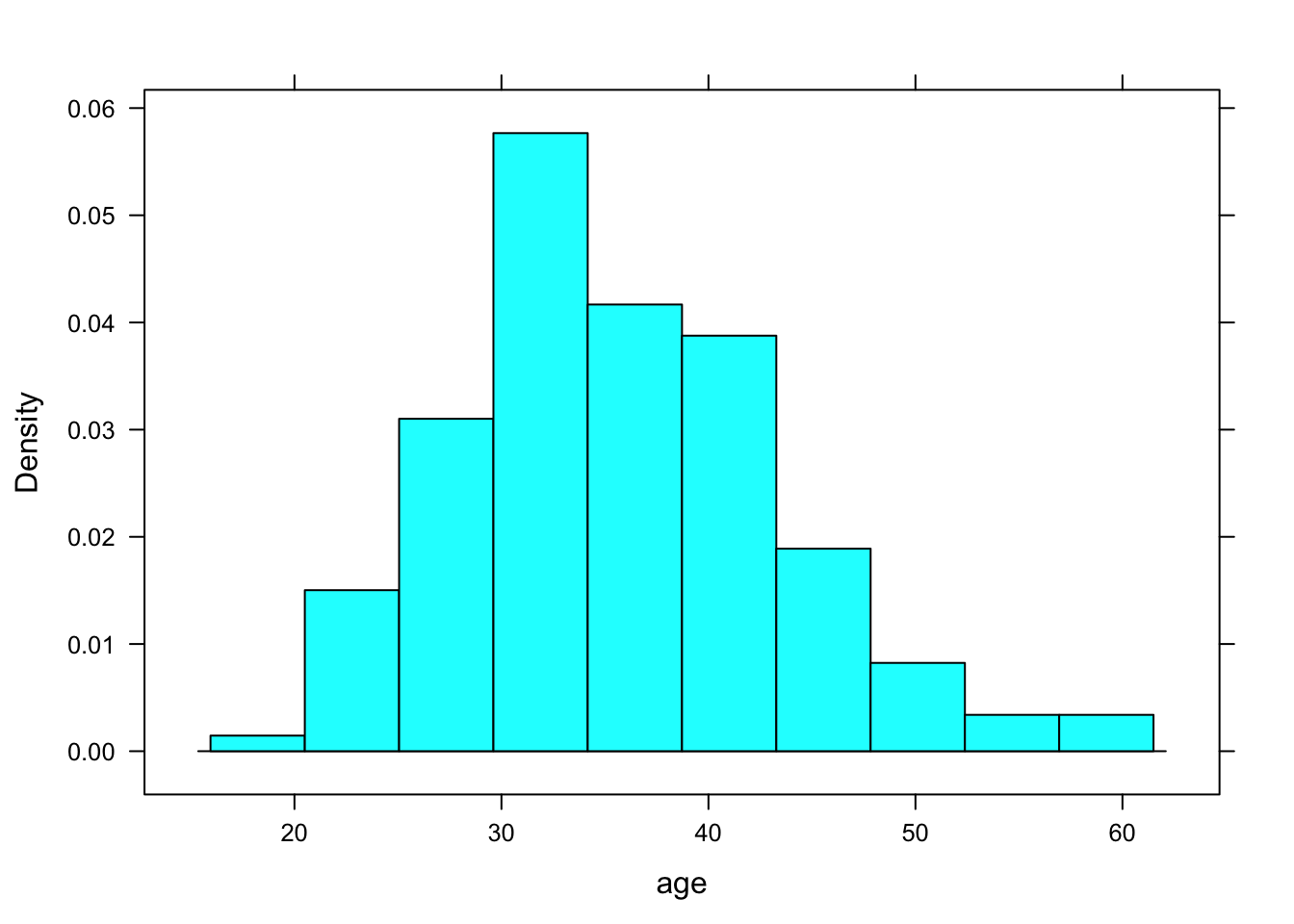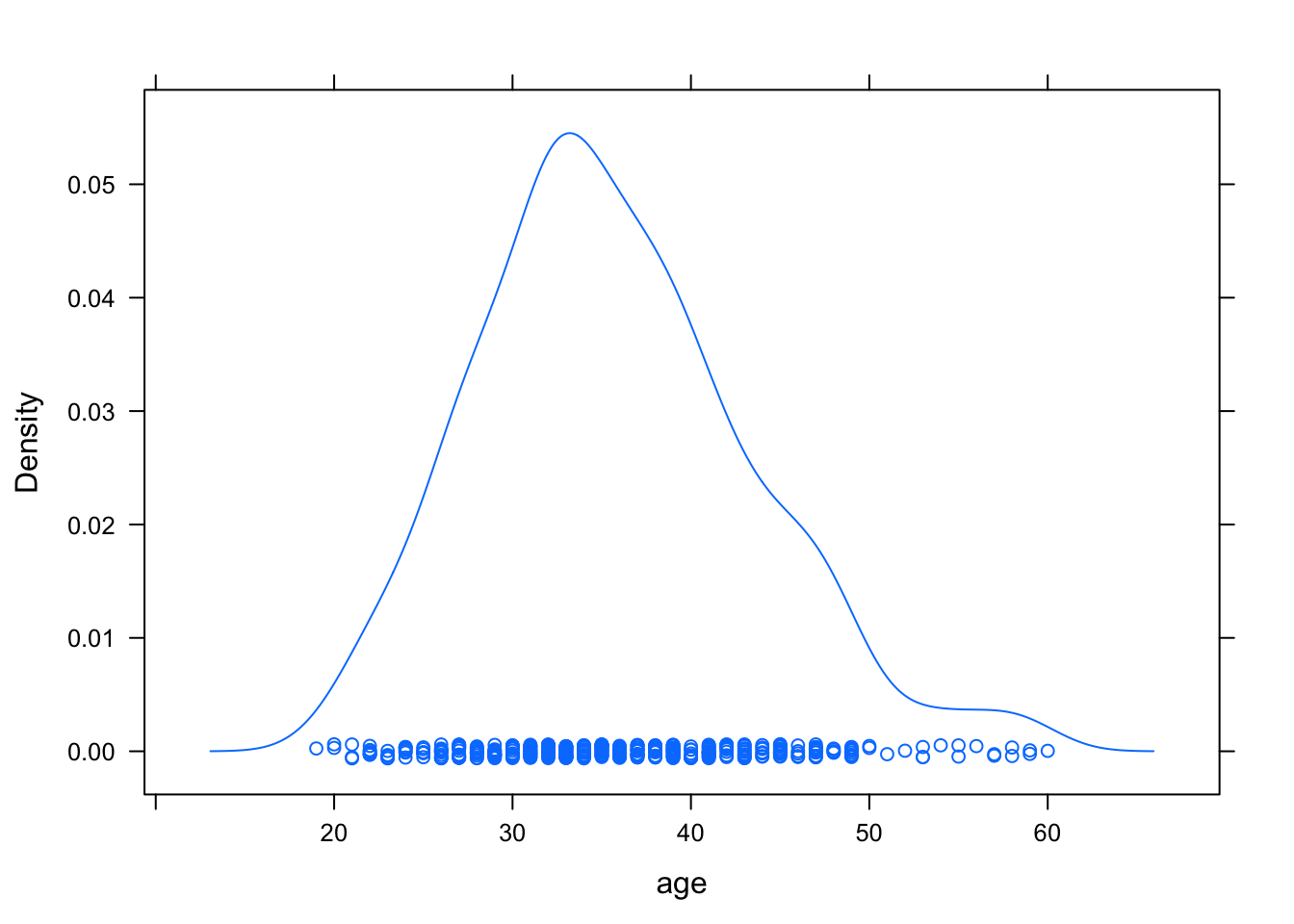This is an R Markdown document. Markdown is a simple formatting syntax for authoring HTML, PDF, and MS Word documents. For more details on using R Markdown see http://rmarkdown.rstudio.com.

When you click the Knit button a document will be generated that includes both content as well as the output of any embedded R code chunks within the document.

### Text

Text can be decorated with bold or italics. It is also possible to

• include mathematics like $$e=mc^2$$ or $y = \beta_0 + \beta_1 x_1 + \beta_2 x_2$

## The most important template is

• goal(y~x,data=mydata)
• What you want R to do is Goal : This determines the function to use(favstat, mean, SD, lm)
• What must R know to do that: This determines the inputs to the function, must identify variables and data frame
• it produces single and multiple variable graphical summaries
• it produces single and multiple variable numerical summaries
• linear models

# Numerical Summaries for one variable

favstats(~ age, data=HELPrct)
##  min Q1 median Q3 max     mean       sd   n missing
##   19 30     35 40  60 35.65342 7.710266 453       0
tally(~ sex, data=HELPrct)
## sex
## female   male
##    107    346

# Graphical Summaries one variable

#graphing quantitative numeric variable
histogram(~age,data=HELPrct)densityplot(~age,data=HELPrct)bwplot(~age,data=HELPrct)